1) Find the number of right angles turned when
(a) The hour hand of a clock goes from 4 to 7.
Ans : 1 right angle.
(b) East and turn anti-clockwise to south.
Ans : 3 right angles.
(c) North and turn to south.

Ans : 2 right angles.
(d) The hour hand of a clock goes from 11 to 5.

Ans : 2 right angles.
(e) Starts at 9 and completes one revolution.

Ans : 4 right angles.

2) What are the degree measures of the following angles?
(a) Straight angle.

Ans : 180˚
(b) Complete angle.

Ans : 360˚
(c) Right angle.

Ans : 90˚

3) Write the angle name for
(a) Half a revolution.

Ans : Straight angle.
(b) One fourth revolution.

Ans : Right angle.
(c) Two one fourth revolution.

Ans : Straight angle.
(d) Less than complete but more than half a revolution.

Ans : Reflex angle.

4) If R,Q,P,S are four points on a line such that QR = 5 cm, RS = 5.5 cm, PQ = 4 cm, SP = 14.5 cm and PR = 9 cm.Which two points of them lies between the other two points.
Ans :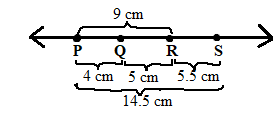Hence point Q and point R his between point P and point S

5) Identify the type of angle from the following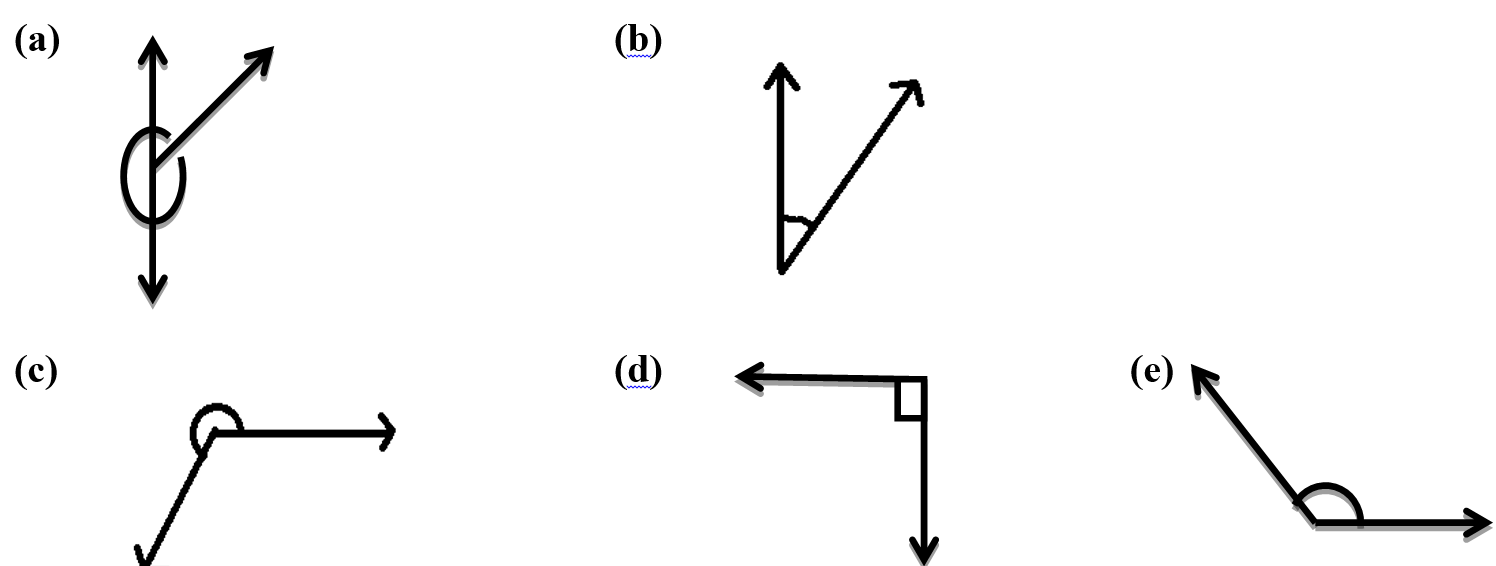Ans :
(a) Straight angle   (b) Acute angle  (c) Reflex angle   (d) Right angle   (e) Obtuse angle.

6) Find the measure of ∠ a in the following :
(1)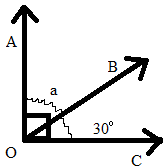(2)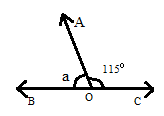(3)(4)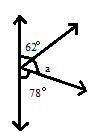Ans :
(1) ∠ a = 90˚− 30˚
(2) ∠ a = 180˚ − 115˚  = 65˚
(3) ∠a = 180˚− 162˚
(4) ∠a = 180˚ − (62˚ + 78˚)

= 180 – 140  = 40˚

7) Identify whether the following are polygons. If not state the reason.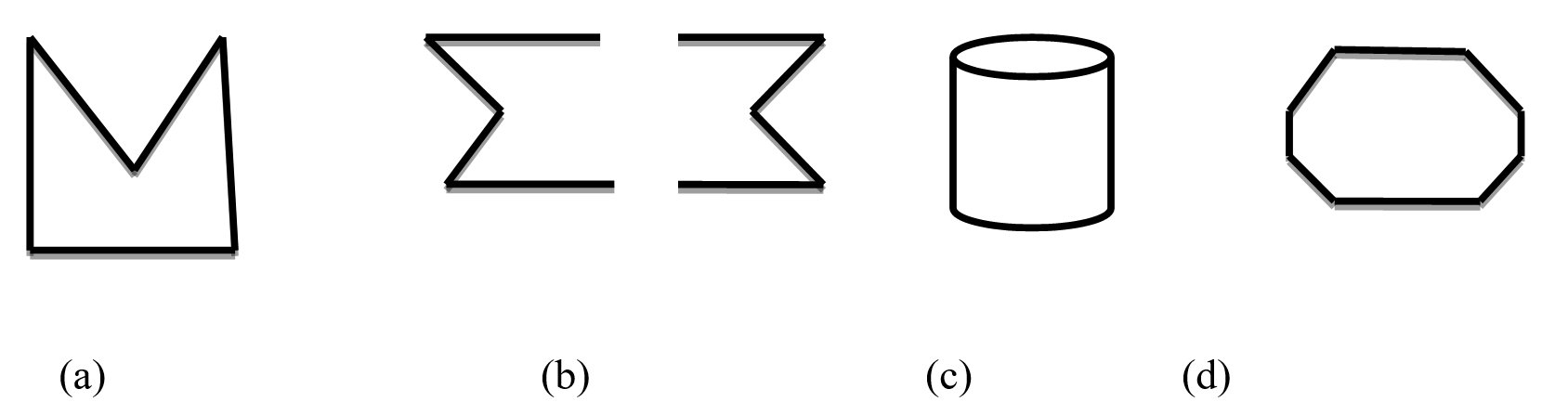Ans :
(a) Polygon .

(b) Not a polygon since it is not closed.
(c) Not a polygon since it is not made up of line segments.
(d) Polygon.

8) Find the measure of  x, y, z in the following :
Ans :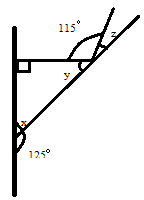(1) m ∠ x = 180 – 125 = 55˚
(2) m ∠ x + m ∠ y + 90˚ = 180˚

m ∠ y = 180 – (90 − ∠ x ) (angle sum property of triangle)
= 180 − (90 − 55)     = 35˚
(3) m ∠z = 180˚ − (115˚ + y)

= 180˚ − (115˚ + 35˚)
= 180˚ − (150˚)    = 30˚

9) Match the following :

 Column I Column II (1) Opposite sides parallel and equal (a) Scalene (2) Unequal sides of triangle (b) Straight angle (3) 8  sided polygon (c) Square (4) Two equal sides of triangle (d) Rhombus (5) Half a revolution (e) Isosceles (6) 4 right angles (f) Parallelogram (g) Octagon

10) State whether true or false :
(1) A cuboid has six faces.

(2) Isosceles triangle has 3 equal sides.
(3) The sum of the measure of all angles of a triangle is 180˚.
(4) A polygon is regular if it’s all sides are equal .
(5) An equilateral triangle is also considered as an isosceles triangle.
(6) The measure of each angle is 90˚in an equilateral triangle.
Ans : (1) True        (2)False         (3) True       (4) False                   (5) False     (6) False